【理论恒叨】【立体匹配系列】经典PatchMatch: （1）Slanted support windows倾斜支持窗模型
【理论恒叨】【立体匹配系列】经典PatchMatch: （2）基于PatchMatch的视差估计
【理论恒叨】【立体匹配系列】经典PatchMatch: （3）后处理（一致性检查与视差填充）

PatchMatchStereo1是Michael Bleyer等在2011年发表于British Machine Vision Conference（BMVC）上的一篇双目立体匹配算法文章，非常经典，倾斜支持窗的思路打破传统固定窗口式局部匹配的思维桎梏，在Middlebury数据集上获得非常好的匹配效果，一段时间内高居排行榜第一名。更难能可贵的是，它和SGM一样数据泛化能力出色，对大部分数据都能取得不错的结果，所以也被很多商业软件所实现，是真正能够产品化的算法。

### 【理论恒叨】【立体匹配系列】经典PatchMatch: （1）Slanted support windows倾斜支持窗模型

• 倾斜支持窗Slanted support windows
• Fronto-parallel windows
• Slanted support windows
• Slanted support windows模型

## 倾斜支持窗Slanted support windows

### Fronto-parallel windows

Fronto-parallel windows是非常经典的窗口模型，它的含义是立体相机正前方与极线纠正后的像平面平行的窗口，同时也和纠正后每个相机的相机坐标系Z轴垂直。该窗口的特性是：

• 窗口内的任意线段在左右图像（核线像对）上的投影长度相等。
• 窗口内的所有空间点的深度一样，由
$D = bf/d$

可知空间点在影像上的投影点视差也相同。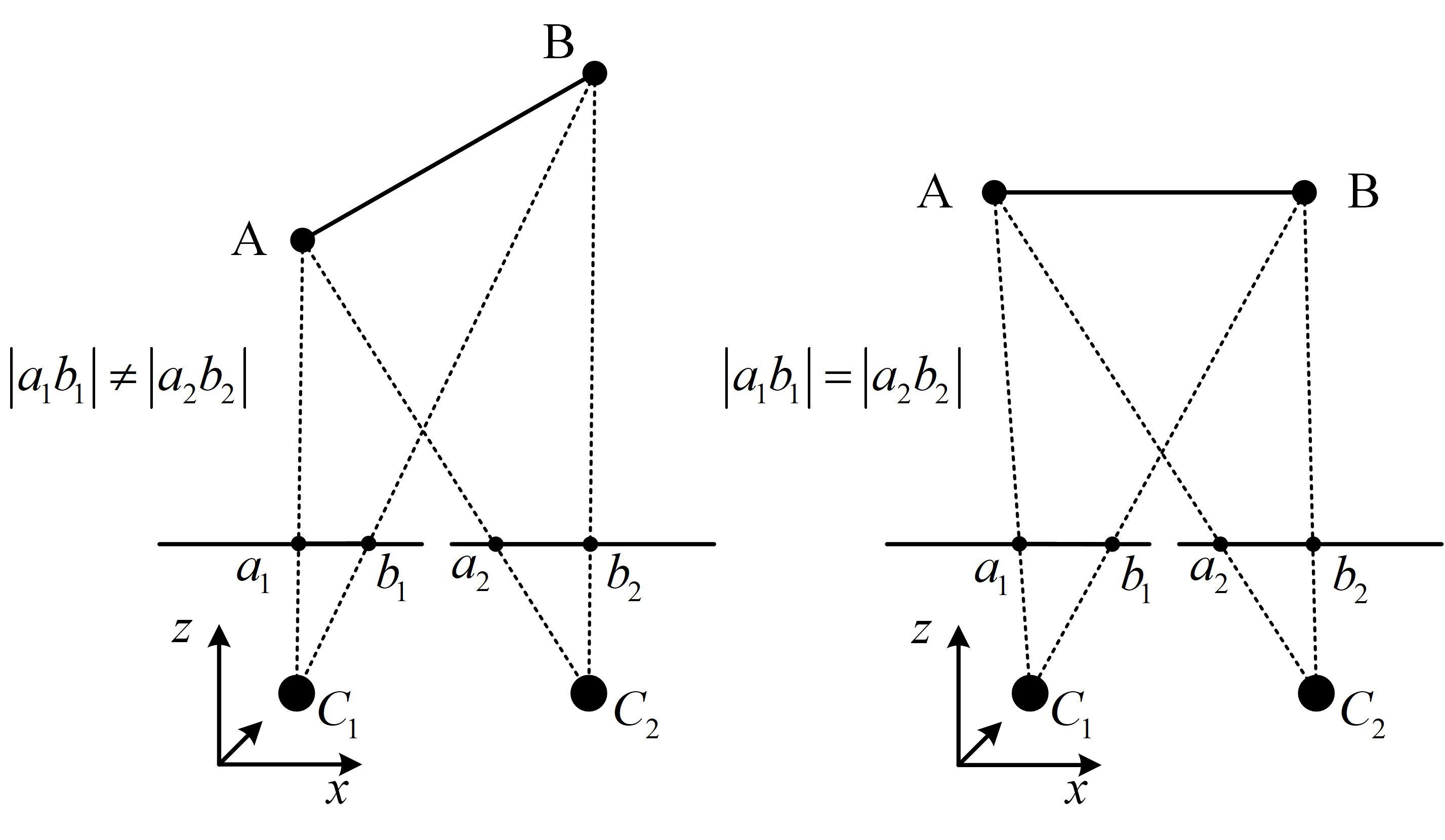### Slanted support windows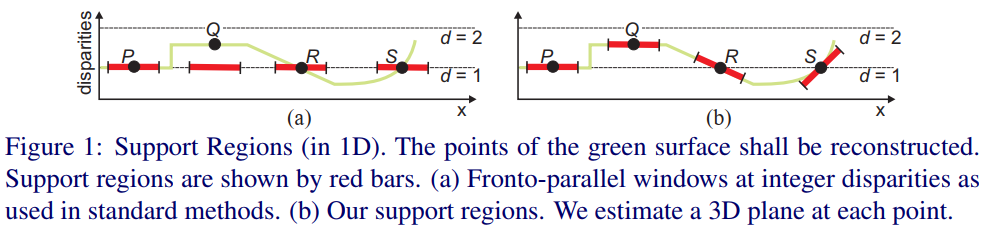## Slanted support windows模型

$p$

$d_p$

，则

$p$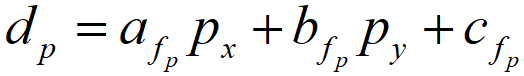$a_{f_p}$

$b_{f_p}$

$c_{f_p}$

$p_x$

$p_y$

$xy$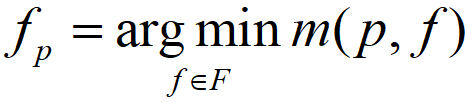$F$

$m(p,f)$

$p$

$f$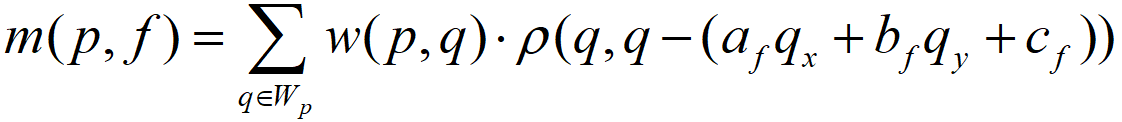$W_p$

$p$

$W_p$

$w(p,q)$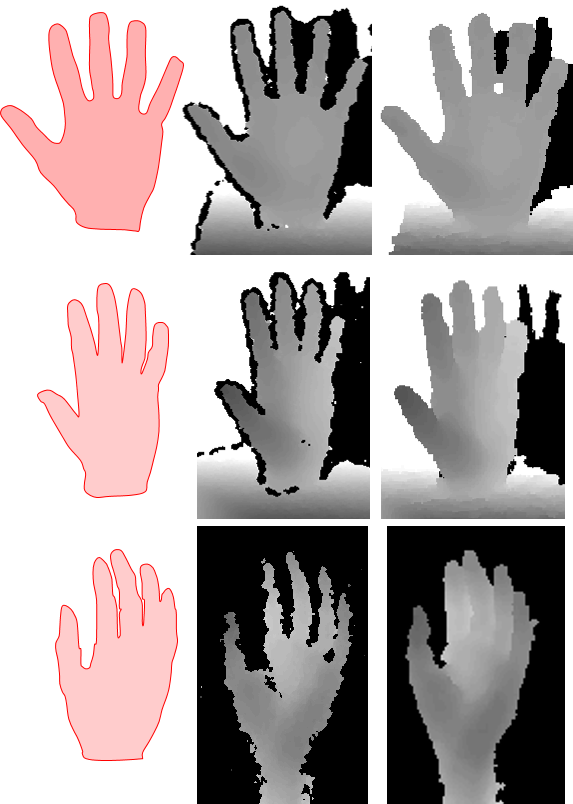PMS计算

$w(p,q)$

$p$

$q$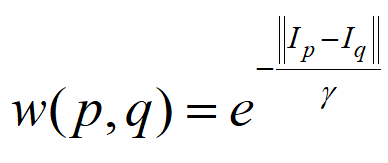$γ$

$，||I_p-I_q||$

$p$

$q$

$|r-r|+|g-g|+|b-b|$

）。相邻像素颜色差异越大，为同一平面的可能性越小，则

$w(p,q)$

$ρ$

。它是在衡量两个像素的不相似性。假设左视图像素

$q$

$a_f$

,

$b_f$

,

$c_f$

)，则它的视差值

$d_q=a_fq_x+b_fq_y+c_f$

，在右视图上对应的同名点

$q'=q-d_q$

$q$

$q'$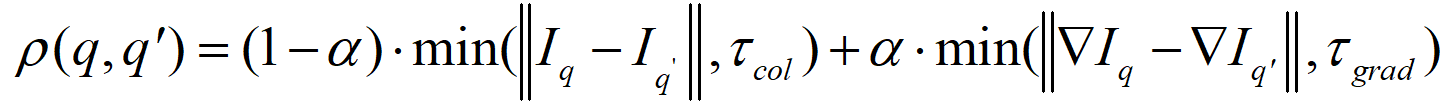$||▽I_p-▽I_{q'}||$

$q$

$q'$

$α$

$q'$

$τ_{col}$

$τ_{grad}$

$p$

$f_p$

$m(p,f)$

，从而可以判定

$f_p$

$f_p$

$F$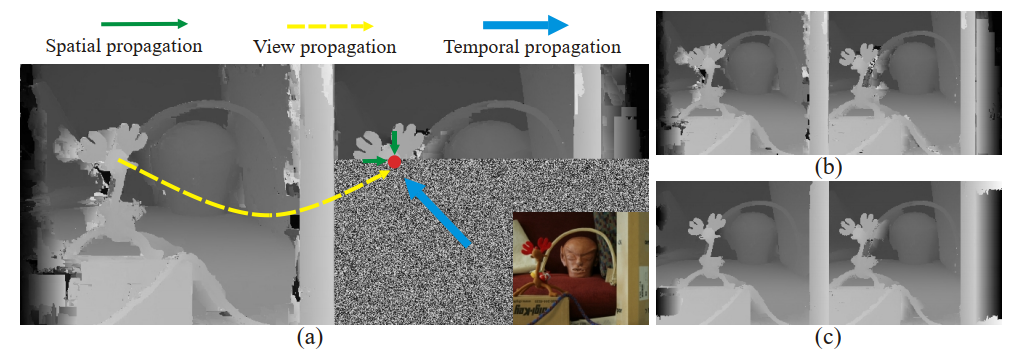【码上实战】【立体匹配系列】经典PatchMatch: （1）框架
【码上实战】【立体匹配系列】经典PatchMatch: （2）主类
【码上实战】【立体匹配系列】经典PatchMatch: （3）随机初始化

Ethan Li 李迎松（知乎：李迎松）

2019年获测绘科技进步一等奖（省部级）

GitHub： https://github.com/ethan-li-coding1. Bleyer M , Rhemann C , Rother C . PatchMatch Stereo - Stereo Matching with Slanted Support Windows[C]// British Machine Vision Conference 2011. ↩︎ ↩︎